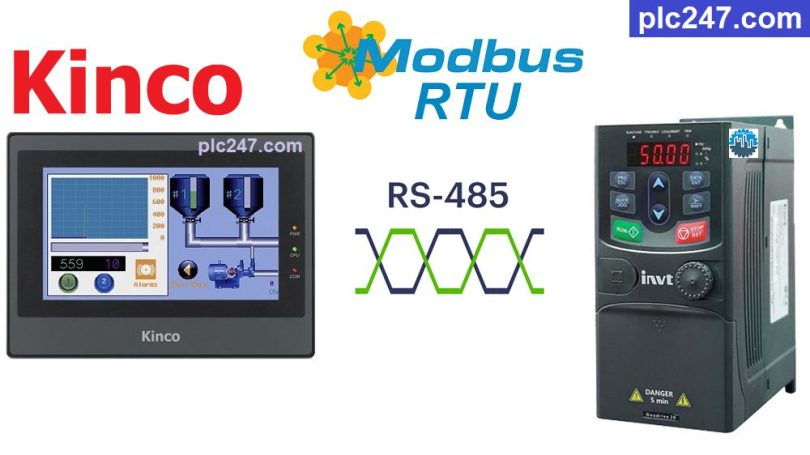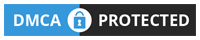# HMI Kinco “Modbus RTU” INVT GD20 TutorialWritten by

GD20 inverter is a new generation multi-function inverter with high performance for general machine building applications. GD20 uses modern open-loop vector control technology with outstanding controllability, can be installed on DIN RAIL with small capacity or on cabinet board.

INVT Goodrive 20 also has a built-in RS485 port which is convenient for controlling and monitoring it with Modbus RTU, today plc247.com will guide you to use HMI Kinco MT4414TE to control it.

#### HMI Kinco & INVT GD20 “Connection Diagram”

+ Using COM.0 port (RS485-2 wire), Kinco HMI models with RS485 ports all have the same connection

#### INVT Goodrive 20 Modbus Parameters Setting

Below are the “Modbus RTU” communication parameters that need to be installed for the Yaskawa V1000 inverter:

• P00.01 = 2 (Frequency Reference via RS485)
• P00.06 = 8 (Motor Control via RS485)
• P00.09 = 0 (“A Frequency” Selection)
• P14.00 = 1 (Slave Address = 1)
• P14.01 = 3 (9600bps)
• P14.02 = 1 (Modbus-RTU 8bit/Even/stop bit = 1)
• P14.03 = 5
• P14.04 = 0.0
##### Note: Kinco HMI Modbus RTU Address = “VFD Address (Dec) + 1“

+ VFD Control Address = 2000 (hex) = 8192 (dec)+ 1 = 8193 (dec)
>>> Set Word “8193” = 1 >>> Forward Run
= 2 >>> Reverse Run
= 5 >>> Stop

+ Frequency Setting Address = 2001 (hex) = 8193 (dec)+ 1 = 8194 (dec)

• Output Frequency Address = 3000 (hex) = 12288 (dec) + 1 = 12289 (dec)
Output Voltage Address = 3003 (hex) = 12291 (dec) + 1 = 12292 (dec)
Output Current Address = 3004 (hex) = 12292 (dec) + 1 = 12293 (dec)

#### HMI Kinco Modbus Parameters Setting

How did we set the Modbus communication parameters for the INVT GD20 inverter, the settings are the same for the Kinco HMI

### HMI Programming

+ Control Panel Design

+ Setting Frequency

+ “Forward Run” Command

+ “Reverse Run” Command

+ “Stop” Command

### Video Tutorial

======

Related Software and Documents